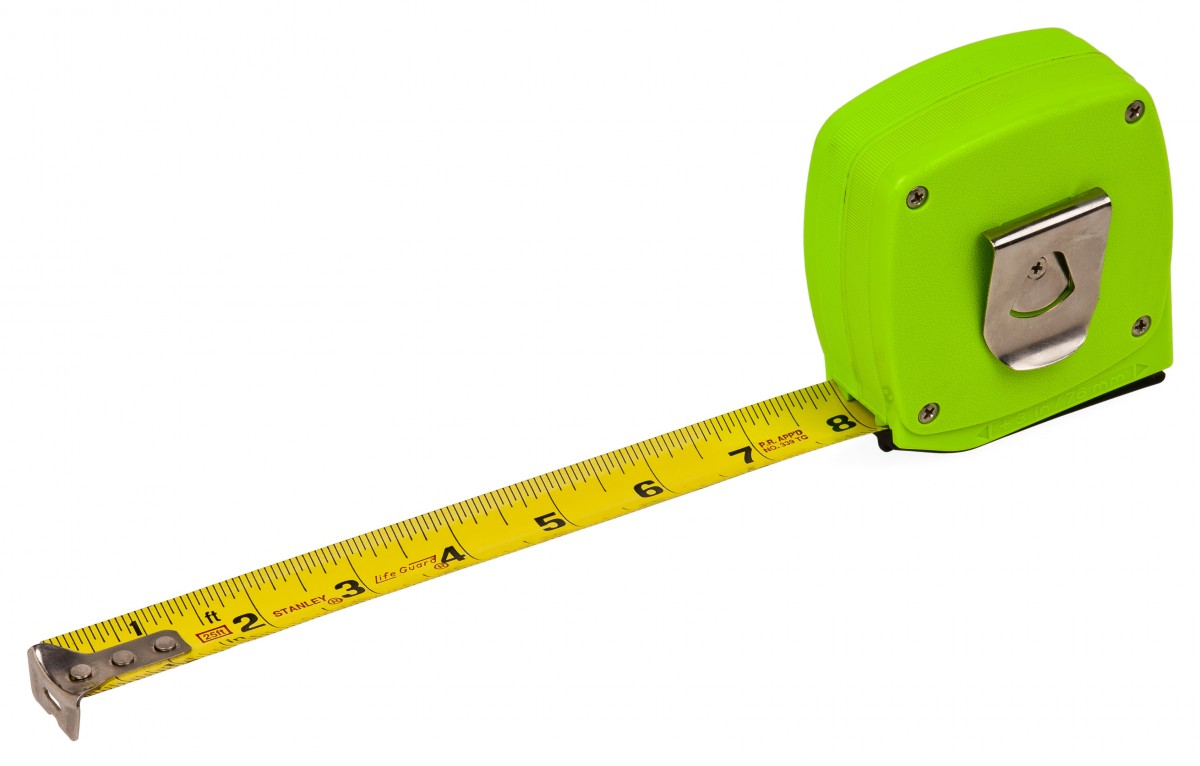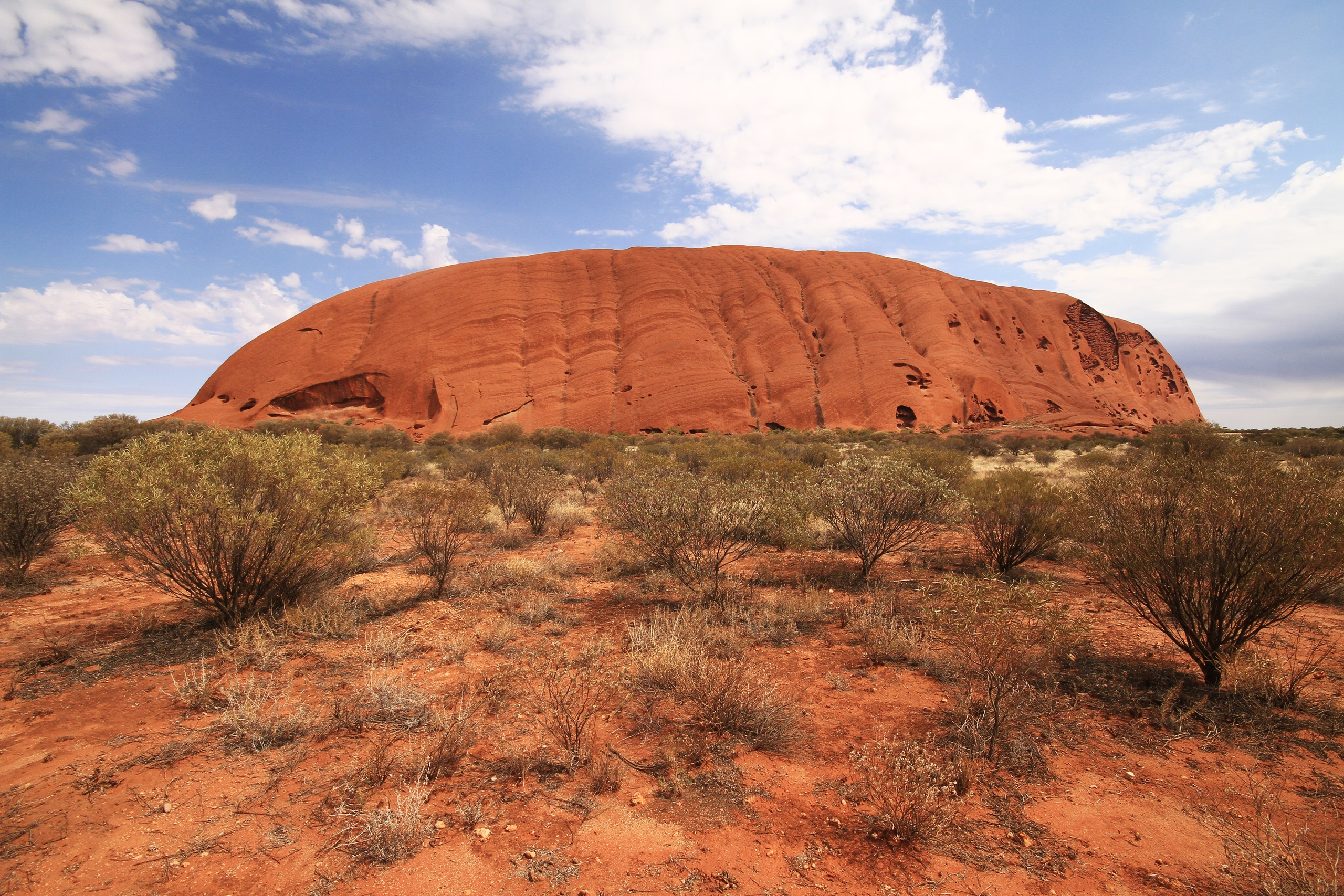# Imperial LengthThis is Blublubox, a friendly alien from the planet BleBeep who is visiting the Earth. He'd like to find out about how Earthlings measure things like heights and distances. These are examples of length measurements.

Well, Blublubox, there are two main systems used for measuring things here on Earth. They are the metric system and the Imperial system (the US standard system is very similar to the Imperial system). In this article, we'll talk about measuring length using the Imperial system. This is the system that the childrens' grandparents might be used to. For lengths, the Imperial units are identical to the US standard units. You can read about the metric system in the article on measuring length in the metric system.

## Units for Length Measurement in the Imperial System

There are many different units used for measuring length in the Imperial system. The most commonly used ones are:

• Inches
• Feet
• Yards
• Miles

### InchesInches are the smallest of the commonly used Imperial units of length. They are used to measure small lengths. Rainfall, the sizes of photographs, and patches for patchwork quilts are measured in inches.

A quick way to estimate a one inch length is to take the length from the top knuckle of your thumb to the tip of your thumb. Of course, everyone's thumbs have different lengths, so this isn't a very accurate measurement.

Some example measurements in inches are:

• A small paper clip is 1 inch long.
• The diameter of a 10 cent piece is just under 1 inch.

To convert from inches to centimetres, remember that one inch is equal to 2.54 centimetres.

### Feet

The foot is the next largest Imperial unit of length:

There are 12 inches in one foot.A foot is the length of an average adult male's sneaker.

Of course, different people have feet of different lengths, so this isn't a very accurate measurement.

Some examples of measurements in feet are

• A humpback whale is $39$-$52$ feet long.
• The average 3 year old child is about 3 feet tall.
• The Sydney Harbour Bridge is 160 feet high• Uluru is 1,142 feet highTo convert from feet to centimetres, remember that one foot is equal to 30.48 centimetres.

### Yards

The yard is the next largest Imperial unit of length:

There are 3 feet in 1 yard. There are also 36 inches in 1 yard.We can use yards (or feet) to measure the lengths of running tracks, playgrounds, rooms in houses and short paths. Fabric is sold by the yard.

Some examples of measurements in yards are

• A 3 year old child is about $1$ yard tall.
• People estimate yards by taking a long step.
• The door knob on your door at home is $1$ yard above the floor.
• The shallow end of a swimming pool is around $1$ yard deep.
• A cricket pitch is $22$ yards long and $3.33$ yards wide.
• The oval at the MCG is $187$ yards long.

To convert from yards to metres, remember that one yard is equal to 0.9144 metres.

### Miles

The mile is the largest of the commonly used Imperial units of length. We use miles to measure long distances.

There are 1,760 yards (5,280 feet) in 1 mile.We measure the distances between cities and the lengths of plane journeys in miles.

Some examples of measurements in miles are

• One mile is the distance you can walk in about $20$ minutes.
• The maximum width of Bass Strait is $217.48$ miles.
• There are $545.3$ miles between Sydney and Melbourne along the M31.
• The Great Barrier Reef is $1,600$ miles long.
• The flight from Sydney to Wellington (NZ) is $1,382$ miles long.

To convert from miles to kilometres, remember that 1 mile is equal to 1.609344 kilometres.

### Conclusion

So, Blublubox, those are the commonly used Imperial measurements. You would measure the width of your spots in inches, your height in feet, the length of the aisle in your space ship in yards, and the diameter of the Earth in miles.

### Description

This mini book covers the core of Math for Foundation, Grade 1 and Grade 2 mathematics including

1. Numbers
3. Subtraction
4. Division
5. Algebra
6. Geometry
7. Data
8. Estimation
9. Probability/Chance
10. Measurement
11. Time
12. Money
13. and much more

This material is provided free of cost for Parent looking for some tricks for their Prekinder, Kinder, Prep, Year 1 and Year 2 children

### Learning Objectives

These lessons are for kids aged 4-8 with the core objective to expose their brains to concepts of addition, subtraction, division, algebra and much more.

Author: Subject Coach
You must be logged in as Student to ask a Question.